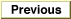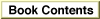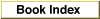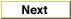# Legacy DocumentImportant: The information in this document is obsolete and should not be used for new development.

Inside Macintosh: QuickDraw GX Environment and Utilities /
Chapter 8 - QuickDraw GX Mathematics / QuickDraw GX Mathematics Reference
Mathematical Functions / Bit Analysis

### FirstBit

You can use the `FirstBit` function to determine the highest order bit that is set in a number.

```short FirstBit (unsigned long x);
```
`x`
The number for which the first bit is to be determined.
function result
The bit number of the highest order bit of the number in the argument.
##### DESCRIPTION
The `FirstBit` function returns the bit number of the highest order bit in a number that is set, or -1 if the number is 0. The highest-order bit is bit 31; the lowest-order bit is bit 0.

##### DESCRIPTION
The use of the `FirstBit` function is described in the section "Analyzing the Bits in a Number" on page 8-33.